# Number Line Plot

The Number Line Plot question type requires students to plot points on a line, or stack points above a line.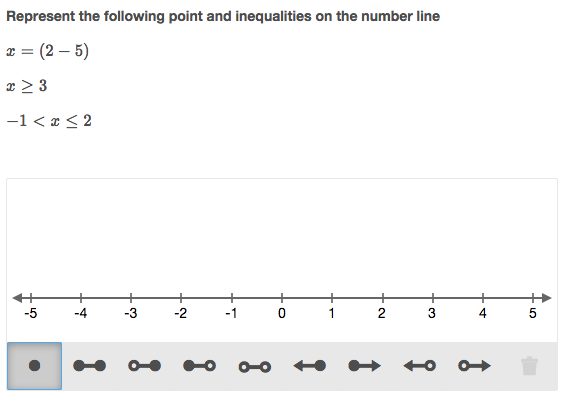Figure 1: Number line plot question example.

## Create a question

Enter the question stem into the Compose question area. Below you will see the Line section where you can configure basic number line parameters.

Add Minimum and Maximum values of the number line. You can also enter the number line title. The title will be displayed underneath the number line unless otherwise specified (see Layout).

Set a value for the Number of responses allowed. This determines the number of elements the user can add to the number line. For instance, if the task is to plot three inequalities the number of responses allowed will be 3.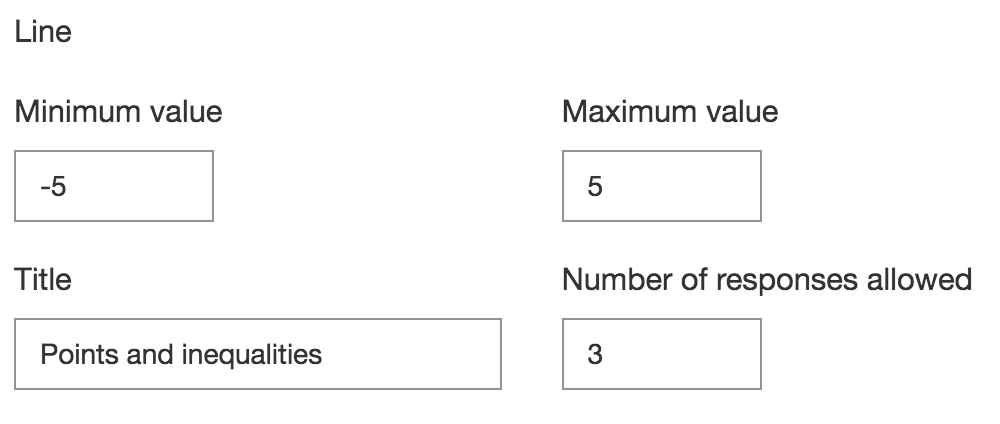Figure 2: Line section settings.

Now configure validation for your question. Follow these steps to set up score and correct response:

• Insert value in the Point(s) box to set up a mark for the question.
• In the Correct tab you will see the number line you just created. In order to validate the question simply plot correct responses on the number line. See example in Figure 3.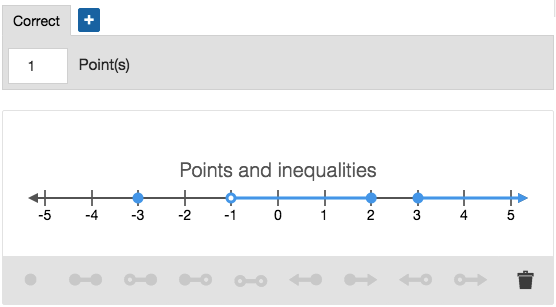Figure 3: Question validation.

## Scoring types

The Scoring type menu can be found under More Options

The following scoring types are available in the Number Line Plot question types:

• Exact Match - Student must answer all parts of the question correct to receive a mark.
• Partial Match Per Response - Each correct response element will be awarded an individual score.
• Partial Match - Each correct response element will be scored individually and the overall question score will be divided between responses.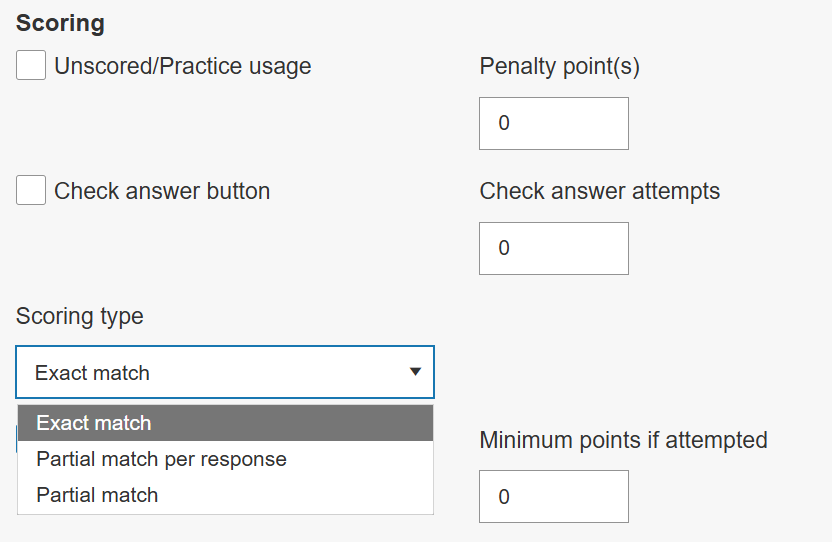Figure 4: Number line plot question scoring types.

## Layout

In this section various styling an be applied to the question. The Layout drop down menu allows authors to decide between horizontal and vertical plotting. You can also add custom Width in pixels. This will set the width of the drawn area.

Line margin (px) defines the distance between the number line extremes and the borders of the drawn area. This should also be given in pixels.

Spacing between stacked responses (px) defines the distance between responses when they are stacked above the number line. The value must be given in pixels.

The Stack responses toggle allows to switch from plotting answers to stacking them above the number line.

Show min arrow and Show max arrow allow to hide or show arrows in the minimum and maximum values of the number line.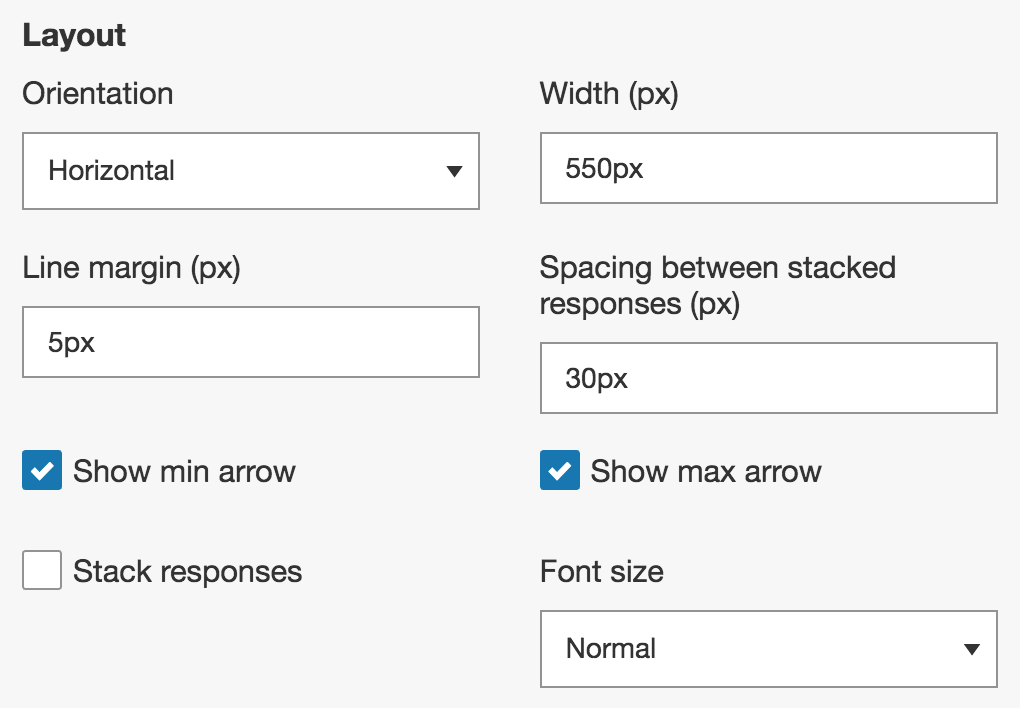Figure 5: Number line layout settings.

## Ticks

This section provides options to configure ticks on the number line.

Show ticks controls the visibility of ticks on the number line.

Rendering base should be defined in case label frequency is greater than 1. This option will determine if labels will render from Zero or Line Minimum Value.

Insert a value in the Distance field - you can insert whole numbers, standard or decimal fractions, or mixed numbers.
You can also specify the value of Minor ticks - minor ticks will be displayed between major ticks.

Show min and Show max toggles control the visibility of the min and max ticks on the number line.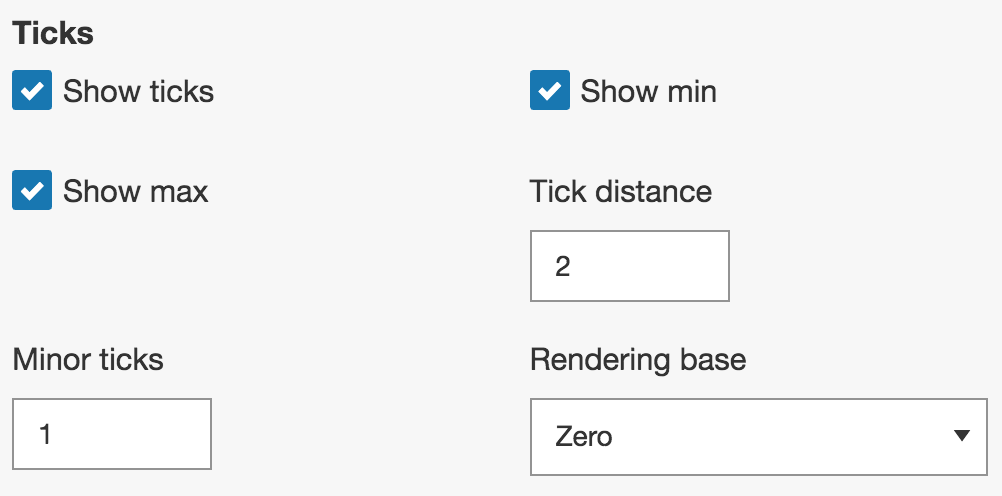Figure 6: Number line ticks settings.

## Labels

Show labels - enable this to show labels on the number line.

Enable Show min and Show max if you want the labels to be drawn on the min and max values of the number line.

You can also add specific points that should be labeled. For this, insert one or more values in the Points field. In case you have more than one value separate them by commas.

## Toolbar

Here you can add or remove buttons that are available for students in the question toolbar. Click on "+Add" below the section title and select the tool from the drop down menu. This will display the tool button in the question toolbar. Click on the bin icon to remove the tool.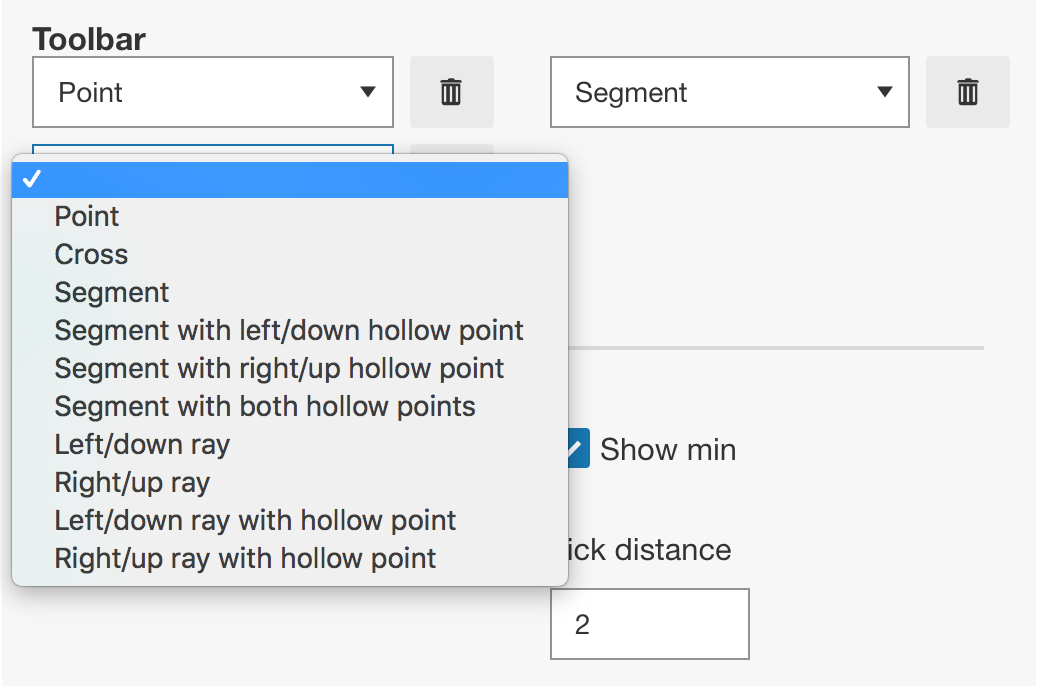Figure 7: Adding tools to the number line.

Following is a list of all tools available in Number Line plot.

Note: there is an alternative cross tool for single point plotting.

 Point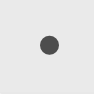Cross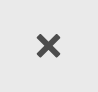Segment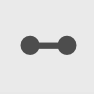Segment with left hollow point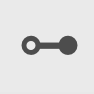Segment with right hollow point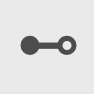Segment with both hollow points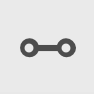Left ray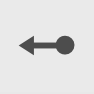Right ray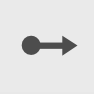Left ray with hollow point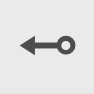Right ray with hollow point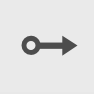DeleteFigure 8: Number line tools.